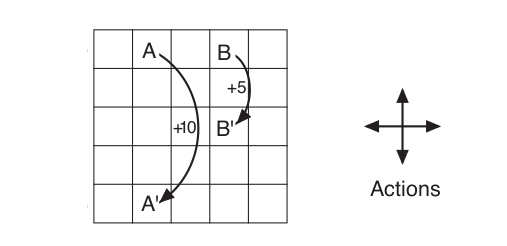# Coding the GridWorld Example from DeepMind’s Reinforcement Learning Course in Python

Source: Deep Learning on MediumSuppose the policy is that the agent selects all four actions with equal probability in all four states. Here in Fig 3.3 the same grid is shown with the State Value Functions for this policy for all states calculated using the following formula (for the discounted reward case equal to 0.9)

If the various tough words like states, state value functions, MDP, reward, policy etc. confuse you , believe me they are much easier than it usually sounds. To learn more about them you should go through David Silver’s Reinforcement Learning Course  or the book “Reinforcement Learning: Second Edition” by Richard S. Sutton and Andrew G. Barto .

So this was all that was given in the example. But I was pretty curious about the real mathematics of how the state value functions of the gridworld were calculated. So I decided to write a python program to calculate them and see if I can get the same values. So lets see my code and how I worked through the problem. ( You can see the full code in my github repo here https://github.com/realdiganta/gridworld)